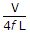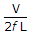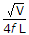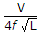# Electronics and Communication Engineering - Power Electronics

### Exercise :: Power Electronics - Section 2

11.

The average load current supplied by a thyristor depends on

 A. firing angle B. firing frequency C. magnitude of gate current D. all of the above

Explanation:

The firing angle controls the period of conduction and hence average load current.

12.

A dc chopper is feeding an RLE load. The maximum steady state ripple is

 A.B.C.D.Explanation:

(Imax - Imin)max =.

13.

In a 3 phase full wave regulator feeding a star connected resistance load the input voltage is 400 V line to line. The firing angle is 160°. The line to line output voltage would be

 A. 400 V B. about 100 V C. about 20 V D. zero

Explanation:

In a three-phase full wave regulator, output voltage is zero if a ≥ 150°.

14.

A thyristor is reverse biased. A positive gate pulse is applied. The thyristor

 A. will be turned on B. may or may not turn on C. will not turn on D. will turn on after sometime

Explanation:

Thyristor can turn on only when it is forward biased.

15.

A single phase series converter is used for

 A. high voltage output B. high current output C. high voltage and high current output D. improving the circuit efficiency